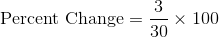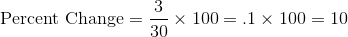# Basic Arithmetic : Decrease

## Example Questions

### Example Question #1 : Finding Percent Change

In March, an athlete weighed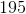pounds. In April, the same athlete weighed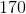pounds. What is the athlete's percentage of weight loss?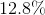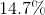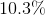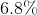Explanation:

The formula to find percentage change is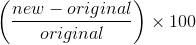.

So for our athlete, our new weight is 170 pounds, and the original weight is 195 pounds.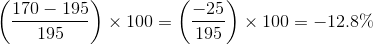Because the question already tells us that we need to find the percent lost, or percent decrease, we can drop the negative sign in the answer.

### Example Question #1 : Finding Percent Change

Last month the shirt you wanted to buy cost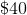and this month it costs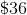. What is the percent decrease?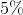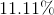Explanation:

1. Subtract the lower price from the higher price.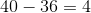2. Divide the difference or amount of change by the initial price: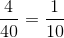or 10% price decrease

### Example Question #3 : Finding Percent Changeis decreased by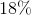. What is the new number?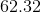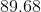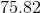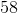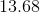Explanation:

We first find 18% of 76: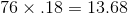Since 76 is being decreased by this amount, we subtract 13.68: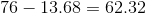### Example Question #1 : Percents And Decimals

At ageyou areinches taller than you wereyears ago, when you wereinches tall. What is the percent change in your height fromyears ago to now? Round your answer to the nearest tenth.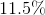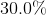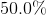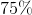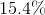Explanation:

The questions throws a lot of numbers at us, but the only ones we are concerned with are those having to do with height. We use the following formula: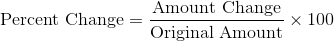We want to know the percent change. We know the amount change, 6 inches, and the original amount, 52 inches. Plugging these into the formula, we get: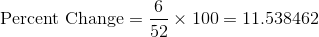Rounding this number to the nearest tenth gives us 11.5%.

### Example Question #4 : Finding Percent Changeis decreased to. What is the percent change?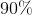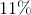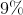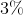Explanation:

Percent change is found by the following formula:Amount change is the difference between the old amount and the new amount.

Here, the original amount is 30, and the new amount is 27. The difference between them is: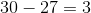We plug in 3 for amount change and 30 for original amount and simplify: